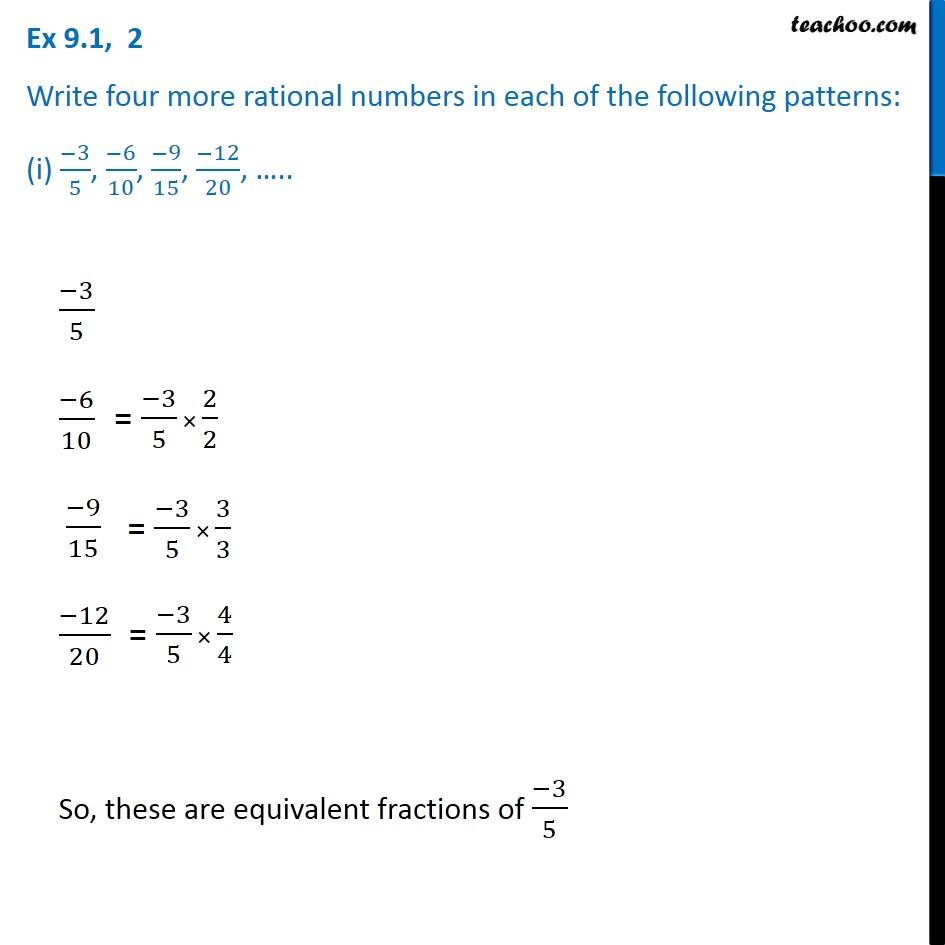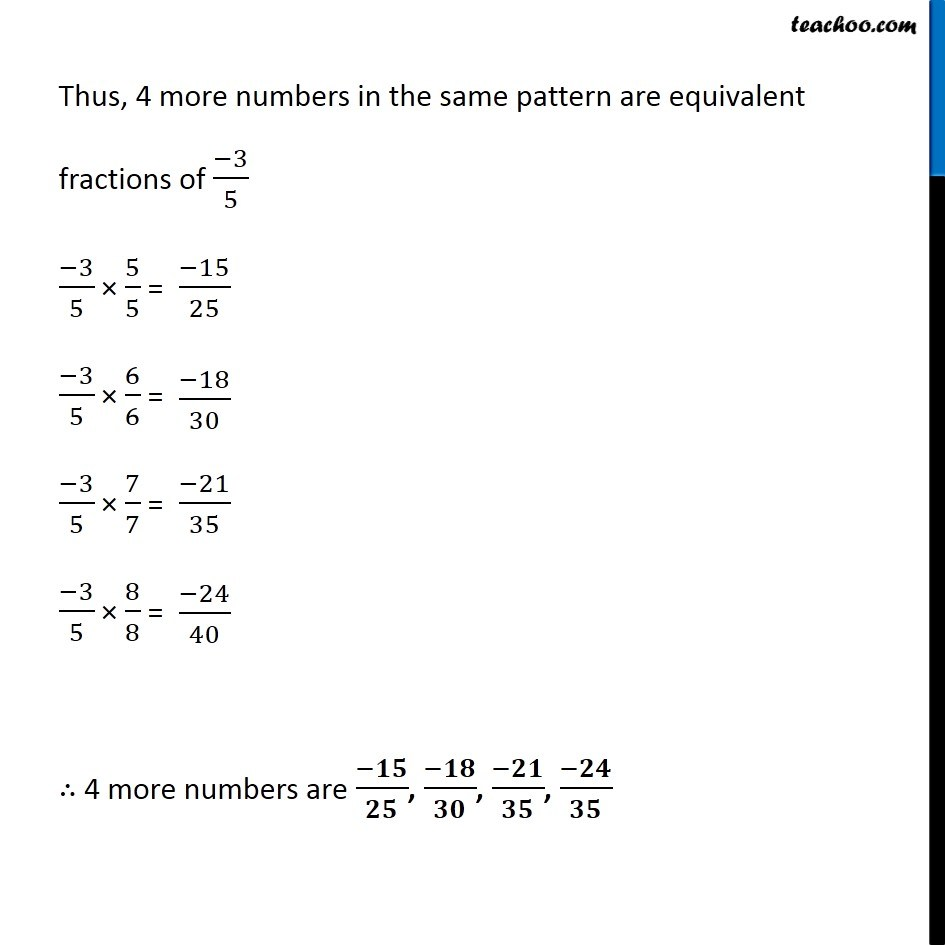1. Chapter 9 Class 7 Rational Numbers
2. Concept wise
3. Equivalent Rational Numbers

Transcript

Ex 9.1, 2 Write four more rational numbers in each of the following patterns: (i) (−3)/5, (−6)/10, (−9)/15, (−12)/20, ….. (−3)/5= (−3)/5 × 2/2 (−6)/10 = (−3)/5 × 3/3 (−9)/15 (−12)/20 = (−3)/5 × 4/4 So, these are equivalent fractions of (−3)/5 Thus, 4 more numbers in the same pattern are equivalent fractions of (−3)/5 (−3)/5 × 5/5 = (−3)/5 × 6/6 = (−3)/5 × 7/7 = (−3)/5 × 8/8 = ∴ 4 more numbers are (−𝟏𝟓)/𝟐𝟓, (−𝟏𝟖)/𝟑𝟎, (−𝟐𝟏)/𝟑𝟓, (−𝟐𝟒)/𝟑𝟓

Equivalent Rational Numbers